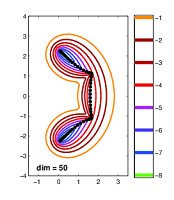# Numerical linear algebraEigenvalue problems are central to a number of applications in science and industry, such as structural dynamics, quantum mechanics, material science and stability analysis. Often the problems are very large and only a few eigenvalues close to a target value are required. Many methods for solving eigenvalue problems iteratively are available, such as the inverse iteration, the Jacobi-Davidson method, and Krylov-methods such as the Lanczos and Arnoldi method. Linear and nonlinear eigenvalue problems that depend on a scalar parameter arise, for example in aerodynamics, electrical power systems and matrix perturbation theory, and are important in determining bifurcations numerically. It is an important research problem to find critical parameter values for parameter dependent eigenproblems such that, for example, eigenvalues coalesce (and become defective, that is, form a Jordan block), eigenvalues cross the imaginary axis (that is, stable matrices become unstable) or move to another critical region in the complex plane.